Olympiad Test Level 1: Length, Weight And Capacity- 1

# Olympiad Test Level 1: Length, Weight And Capacity- 1

Test Description

## 15 Questions MCQ Test Mathematics Olympiad for Class 1 | Olympiad Test Level 1: Length, Weight And Capacity- 1

Olympiad Test Level 1: Length, Weight And Capacity- 1 for Class 1 2023 is part of Mathematics Olympiad for Class 1 preparation. The Olympiad Test Level 1: Length, Weight And Capacity- 1 questions and answers have been prepared according to the Class 1 exam syllabus.The Olympiad Test Level 1: Length, Weight And Capacity- 1 MCQs are made for Class 1 2023 Exam. Find important definitions, questions, notes, meanings, examples, exercises, MCQs and online tests for Olympiad Test Level 1: Length, Weight And Capacity- 1 below.
 1 Crore+ students have signed up on EduRev. Have you?
Olympiad Test Level 1: Length, Weight And Capacity- 1 - Question 1

### Which of the following holds a maximum amount of water?

Detailed Solution for Olympiad Test Level 1: Length, Weight And Capacity- 1 - Question 1

Glass (a) hold the maximum amount of water.

Olympiad Test Level 1: Length, Weight And Capacity- 1 - Question 2

### Which balloon has the shortest string?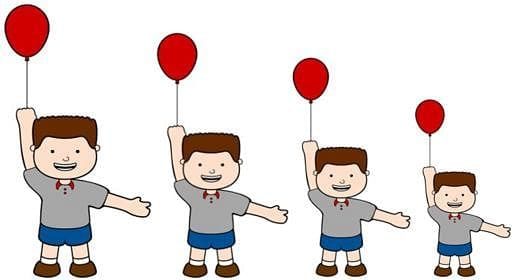Detailed Solution for Olympiad Test Level 1: Length, Weight And Capacity- 1 - Question 2

Balloon D has the shortest string.

Olympiad Test Level 1: Length, Weight And Capacity- 1 - Question 3

### Which is the smallest rectangle?

Detailed Solution for Olympiad Test Level 1: Length, Weight And Capacity- 1 - Question 3

Rectangle (c) is the smallest rectangle.

Olympiad Test Level 1: Length, Weight And Capacity- 1 - Question 4

Which is the longest bar?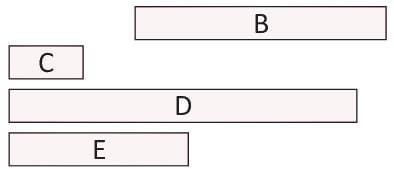Detailed Solution for Olympiad Test Level 1: Length, Weight And Capacity- 1 - Question 4

Bar D is the longest ball.

Olympiad Test Level 1: Length, Weight And Capacity- 1 - Question 5

The lightest among the following is

Detailed Solution for Olympiad Test Level 1: Length, Weight And Capacity- 1 - Question 5

The flower is the lightest.

Olympiad Test Level 1: Length, Weight And Capacity- 1 - Question 6

Which of the following is the measuring unit of length?

Detailed Solution for Olympiad Test Level 1: Length, Weight And Capacity- 1 - Question 6

Centimetre is the measuring unit of length.

Olympiad Test Level 1: Length, Weight And Capacity- 1 - Question 7

Which one of the following holds the least amount of water?

Detailed Solution for Olympiad Test Level 1: Length, Weight And Capacity- 1 - Question 7

Glass (d) holds least amount of water.

Olympiad Test Level 1: Length, Weight And Capacity- 1 - Question 8

Which of the following is the measuring unit of weight?

Detailed Solution for Olympiad Test Level 1: Length, Weight And Capacity- 1 - Question 8

Gram is the measuring unit of weight

Olympiad Test Level 1: Length, Weight And Capacity- 1 - Question 9

The length of the ruler is measured in

Detailed Solution for Olympiad Test Level 1: Length, Weight And Capacity- 1 - Question 9

The length of the ruler is measured in centimeter.

Olympiad Test Level 1: Length, Weight And Capacity- 1 - Question 10

The length of penguin is equal to ____________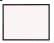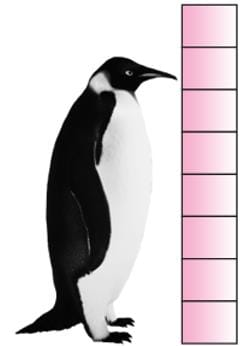Detailed Solution for Olympiad Test Level 1: Length, Weight And Capacity- 1 - Question 10

The length of penguin is equal to 7

Olympiad Test Level 1: Length, Weight And Capacity- 1 - Question 11

Which of the following cup is longer than D?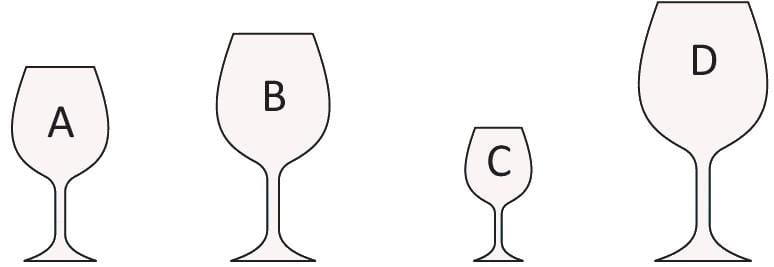Detailed Solution for Olympiad Test Level 1: Length, Weight And Capacity- 1 - Question 11

None of the cup is longer than D

Olympiad Test Level 1: Length, Weight And Capacity- 1 - Question 12

What is the length of the tape?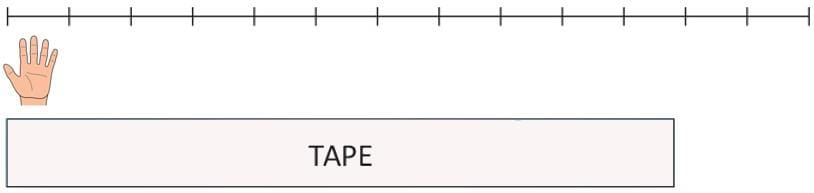Detailed Solution for Olympiad Test Level 1: Length, Weight And Capacity- 1 - Question 12

The length of the tape is 12 span.

Olympiad Test Level 1: Length, Weight And Capacity- 1 - Question 13

The heaviest among the following is.

Detailed Solution for Olympiad Test Level 1: Length, Weight And Capacity- 1 - Question 13

The heaviest is Elephant.

Olympiad Test Level 1: Length, Weight And Capacity- 1 - Question 14

Which of the following object is thicker than your water bottle?

Detailed Solution for Olympiad Test Level 1: Length, Weight And Capacity- 1 - Question 14

The bucket is thicker than your water bottle.

Olympiad Test Level 1: Length, Weight And Capacity- 1 - Question 15

The length of the axe is equal to _________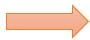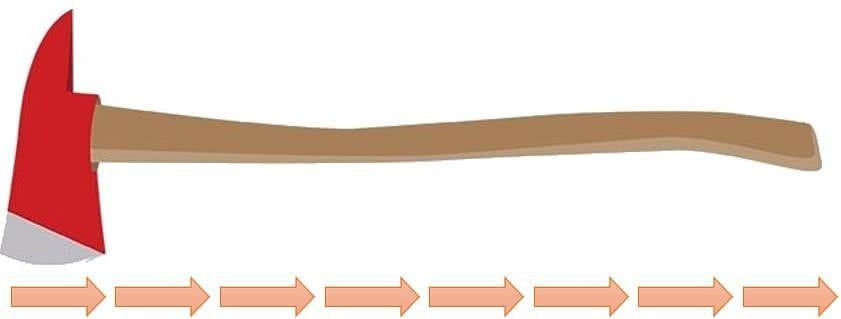Detailed Solution for Olympiad Test Level 1: Length, Weight And Capacity- 1 - Question 15

The length of the axe is equal to 8

## Mathematics Olympiad for Class 1

9 docs|51 tests
 Use Code STAYHOME200 and get INR 200 additional OFF Use Coupon Code
Information about Olympiad Test Level 1: Length, Weight And Capacity- 1 Page
In this test you can find the Exam questions for Olympiad Test Level 1: Length, Weight And Capacity- 1 solved & explained in the simplest way possible. Besides giving Questions and answers for Olympiad Test Level 1: Length, Weight And Capacity- 1, EduRev gives you an ample number of Online tests for practice

9 docs|51 tests